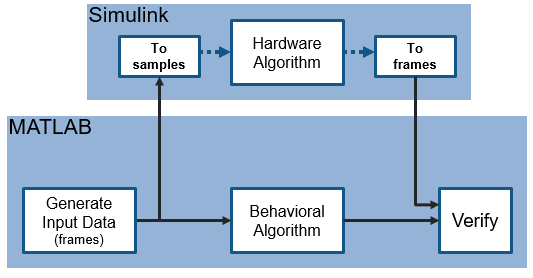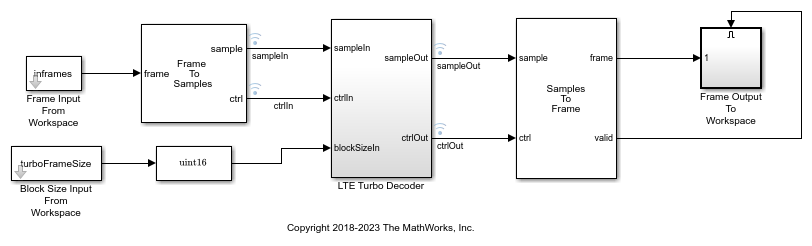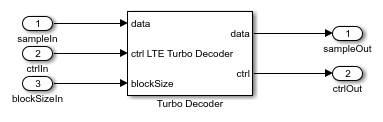Main Content

# Verify Turbo Decoder with Framed Data from MATLAB

This example shows how to verify a hardware-targeted LTE Turbo Decoder design using frames of data from MATLAB®.

To run this example, use the VerifyLTEHDLTurboDecoderFramedData.m script.

LTE Toolbox™ and 5G Toobox™ functions model operations on framed, floating-point and integer data and provide excellent behavioral references. Hardware designs must use streaming Boolean or fixed-point data. This example imports framed data to Simulink® and then converts to a sample stream for hardware algorithm design. The same data is applied to both the hardware algorithm in Simulink, and the behavioral algorithm in MATLAB. The Simulink model converts the output sample stream to frames and exports the frames to MATLAB for comparison.### Hardware Targeting in Simulink

The key features of a model for hardware targeting in Simulink® are:

• Streaming Sample Interface: LTE Toolbox and 5G Toolbox functions process frames while blocks in Wireless HDL Toolbox use a streaming sample interface. Serial processing is efficient for hardware designs. For further information, see Streaming Sample Interface. You can convert frames to samples in Simulink using the Frame To Samples block or in MATLAB using the `whdlFramesToSamples` function. In this example, we convert the frames to a stream of samples in Simulink using the Frame To Samples block.

• Subsystem Targeted for HDL Code Generation: Design a hardware-friendly sample-streaming model by selecting blocks from the Blocks. The part of the design targeted for HDL code generation must be in a separate subsystem.

• Conversion of Sample-based Output To Frames: For verification, you can export the result of your hardware-compatible design to the MATLAB® workspace. You can then compare this result with the output of a MATLAB behavioral design. In this example, we convert the samples to frames in Simulink using the Samples To Frame block.

You can use the VerifyLTEHDLTurboDecoderFramedData.m MATLAB script to run the MATLAB behavioral code, set up, import data and run the Simulink™ model, export the data, and compare the behavioral and Simulink output.

The MATLAB script contains four parts:

1. Behavioral Simulation of Turbo Decoder

2. Set Up the Simulink Model for Hardware Design

3. Run Simulink Model

4. Verify Output of Simulink Model

### Behavioral Simulation of Turbo Decoder

For a behavioral simulation of the design, use the `lteTurboDecode` function from LTE Toolbox. This code generates input data, softBits that we can use as input for the HDL targeted design. The behavioral output of the `lteTurboDecode` function, rxBits can be used for comparison to the output of the HDL targeted design. Both softBits and rxBits are frames of floating-point data.

```% Turbo decoding of soft bits obtained from a noisy constellation turboFrameSize = 6144; txBits = randi([0 1],turboFrameSize,1); codedData = lteTurboEncode(txBits); txSymbols = lteSymbolModulate(codedData,'QPSK'); noise = 0.5*complex(randn(size(txSymbols)),randn(size(txSymbols))); rxSymbols = txSymbols + noise; scatter(real(rxSymbols),imag(rxSymbols),'co'); hold on; scatter(real(txSymbols),imag(txSymbols),'rx') legend('Rx constellation','Tx constellation') softBits = lteSymbolDemodulate(rxSymbols,'QPSK','Soft'); rxBits = lteTurboDecode(softBits); ```

### Set Up the Simulink Model for Hardware Design

The model imports frame-based data from the MATLAB workspace. In this case, the variable inframes and blockSizeIn are the inputs to the model.The LTE Turbo Decoder subsystem consists of the LTE Turbo Decoder block. This block is set to run for a frame size of 6144 systematic bits and 6 iterations of decoding.The Simulink model imports frames of data from MATLAB and converts them into a stream of samples in the Simulink model.

The Frame To Samples block converts the framed data to a stream of samples and control signals. This block also adds invalid samples to the sample stream to mimic streaming hardware data. This block provides the input to the subsystem targeted for HDL code generation, but does not itself support HDL code generation.

In this example, the Frame To Samples block is configured to provide the input to the LTE Turbo Decoder block. No invalid samples are inserted between valid samples.

The Simulink model also imports the frame size parameter from the workspace and passes it to the blockSize port of the LTE Turbo Decoder block. This example uses one block size and so this port remains at a constant value specified by the turboFrameSize parameter.

The LTE Turbo Decoder block takes in one frame of data, runs the specified number of iterations, and can then accept another input frame. In order to allow the block processing time to run the 6 iterations, we send invalid samples between frames. In this example, we set the number of invalid samples between frames to 7 iterations (or 14 half-iterations, each of which corresponds to a frame of delay). To calculate the exact cycles needed to process a frame, look at the LTE Turbo Decoder.

The turbo encoder function sends all systematic bits out first, followed by the first set of parity bits and finally, the second set of parity bits. The LTE Turbo Decoder however requires each set of systematic and 2 parity bits to be sent in together. To reshape the input data to the LTE Turbo Decoder block accordingly, we choose the option to compose the output samples from interleaved input samples.

```% Open the model modelname = 'TurboDecoderFramedDataHDLExample'; open_system(modelname); ```
```% Settings for Frame Input From Workspace block inframesize = length(softBits); ```
```% Setting for LTE Turbo Decoder block numTurboIterations = 6; ```
```% Settings for Frame To Samples block idlecyclesbetweensamples = 0; % approximate latency from LTE Turbo Decoder block per half-iteration tdlatency = (ceil(turboFrameSize/32)+4)*32; % approximate delay in frames for LTE Turbo Decoder output algframedelay = (2*numTurboIterations + 2)*(ceil(tdlatency/turboFrameSize)); % Rate 1/3 code: Systematic + 2 Parity insamplesize = 3; idlecyclesbetweenframes = (inframesize/insamplesize)*algframedelay; % Select the option to compose output from interleaved input samples set_param([modelname '/Frame To Samples'],... 'InterleaveSamples','on'); ```
```% Settings for Samples To Frame block % Exact setting, includes idle cycles introduced totalframesize = ((inframesize/insamplesize)*... (idlecyclesbetweensamples+1))+idlecyclesbetweenframes; % Alternative setting, same size as LTE Turbo Decoder output frame % totalframesize = outframesize; outsamplesize = 1; ```

The MATLAB code also converts the input data to fixed-point for use in the hardware-targeted model, and computes the desired simulation time.

```% Simulation time numFrames = 1; % number of frames to run simTime = numFrames; ```
```% Input frame data for model % use same data as lteTurboDecode, converted to fixed point inframes = fi(softBits, 1, 5, 2); ```

### Run Simulink Model

You can run the model by clicking the Play button or calling the sim command on the MATLAB command line.

```sim(modelname); ```

### Verify Output of Simulink Model

Running the model results in frames of data logged from the Frame Output To Workspace block in the variable outframes_logdata. Compare the data to the output of the behavioral simulation to find the number of bit mismatches between the behavioral code and hardware-targeted model.

This simulation results in no bit errors. Increasing the amount of noise added to the samples or reducing the number of iterations may result in bit errors.

```% Process output of HDL model and compare to behavioral simulation output % Gather all the logged data into one array rxBits_hdl = outframes_logdata(:); ```
```% Check number of bit mismatches numBitsDiff = sum(rxBits_hdl ~= rxBits); fprintf(['\nLTE Turbo Decoder: Behavioral and ' ... 'HDL simulation differ by %d bits\n\n'], numBitsDiff); ```### Generate HDL Code and Verify Its Behavior

Once your design is working in simulation, you can use HDL Coder™ to Generate HDL Code for the LTE Turbo Decoder subsystem. Use HDL Verifier™ to generate a SystemVerilog DPI Test Bench (HDL Coder) or run FPGA-in-the-Loop.

```makehdl([modelname '/LTE Turbo Decoder']) % Generate HDL code makehdltb([modelname '/LTE Turbo Decoder']) % Generate HDL Test bench ```

## Support

#### Deploying 5G NR Wireless Communications on FPGAs: A Complete MATLAB and Simulink Workflow

Download white paper